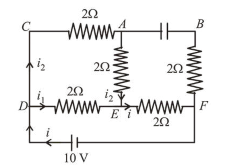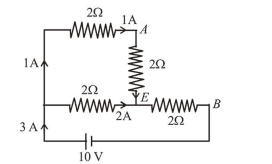# An ideal cell of emf 10 V is connected in circuit shown in figure.Question:

An ideal cell of emf $10 \mathrm{~V}$ is connected in circuit shown in figure. Each resistance is $2 \Omega$. The potential difference (in V) across the capacitor when it is fully charged is _______Solution:As capacitor is fully charged no current will flow through it.We have the current distribution as shown in the figure.

Equivalent resistance, $\mathrm{R}_{\mathrm{eq}}=\left(\frac{4 \times 2}{4+2}\right)+2$

Net current, $i=\frac{10}{\frac{4}{3}+2}=\frac{10 \times 3}{10}=3$ Amp

$i_{1}=2 \mathrm{~A}$ and $i_{2}=1 \mathrm{~A}$

$V_{A E B}=1 \times 2+3 \times 2=8 \mathrm{~V}$